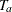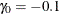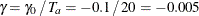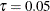# The SEQDESIGN Procedure

### Example 101.15 Computing Sample Size for Survival Data with Truncated Exponential Accrual

This example illustrates sample size computation for survival data when the accrual is not uniform and when the data contain individual loss to follow-up.

Following the derivations in Example 101.14, the following statements invoke the SEQDESIGN procedure for sample size computation with exponential accrual and individual loss to follow-up:

proc seqdesign;
OBrienFleming: design nstages=4 method=obf
;
samplesize model=twosamplesurvival
( nullhazard=0.03466
hazard=0.01733
accrual=exp(parm=-0.1)
loss=exp(hazard=0.05)
acctime=20);
run;


The SAMPLESIZE statement derives required sample sizes for the test. The MODEL=TWOSAMPLESURVIVAL option specifies a log-rank test to compare two survival distributions for the treatment effect. The NULLHAZARD=0.03466 option specifies null hazard rates for the two groups under the null hypothesis, and the HAZARD=0.01733 option specifies the hazard rate for the first group under the alternative hypothesis.

The ACCTIME= option specifies the accrual time, and the ACCRUAL=EXP(POWER=–0.1) option specifies that the individual accrual be truncated exponential with a scaled power parameter. That is, the truncated exponential accrual has a power parameterin the accrual time. The LOSS=EXP(HAZARD=0.05) option specifies an exponential loss function with a hazard rate. For a detailed derivation of these required sample sizes, see the section Test for Two Survival Distributions with a Log-Rank Test.

The "Design Information" table in Output 101.15.1 displays design specifications and the derived maximum information.

The SEQDESIGN Procedure
Design: OBrienFleming

Statistic Distribution Normal
Boundary Scale Standardized Z
Alternative Hypothesis Two-Sided
Early Stop Reject Null
Method O'Brien-Fleming
Boundary Key Both
Alternative Reference 0.693147
Number of Stages 4
Alpha 0.05
Beta 0.1
Power 0.9
Max Information (Percent of Fixed Sample) 102.2163
Max Information 22.35451
Null Ref ASN (Percent of Fixed Sample) 101.5728
Alt Ref ASN (Percent of Fixed Sample) 76.7397

The "Boundary Information" table in Output 101.15.2 displays information level, alternative reference, and boundary values.

Output 101.15.2: Boundary Information

Boundary Information (Standardized Z Scale)
Null Reference = 0
_Stage_   Alternative Boundary Values
Information Level Reference Lower Upper
Proportion Actual Events Lower Upper Alpha Alpha
1 0.2500 5.588627 22.35451 -1.63862 1.63862 -4.04859 4.04859
2 0.5000 11.17725 44.70902 -2.31736 2.31736 -2.86278 2.86278
3 0.7500 16.76588 67.06352 -2.83817 2.83817 -2.33745 2.33745
4 1.0000 22.35451 89.41803 -3.27724 3.27724 -2.02429 2.02429

The "Sample Size Summary" table in Output 101.15.3 displays parameters for the sample size computation. Because the ACCNOBS= option is not specified along with the ACCTIME= option, the minimum and maximum sample sizes are derived.

Output 101.15.3: Sample Size Summary

Sample Size Summary
Test Two-Sample Survival
Null Hazard Rate 0.03466
Hazard Rate (Group A) 0.01733
Hazard Rate (Group B) 0.03466
Hazard Ratio 0.5
log(Hazard Ratio) -0.69315
Reference Hazards Alt Ref
Accrual Truncated Exponential with Losses
Scaled Exponential Parameter -0.1
Loss Hazard Rate 0.05
Accrual Time 20
Min Accrual Sample Size 269
Max Accrual Sample Size 553
Max Number of Events 89.41803

With the minimum and maximum sample sizes of 269 and 553, respectively, the ACCNOBS=360 option specifies an accrual sample size of 360 for the trial.

proc seqdesign;
OBrienFleming: design nstages=4
method=obf
;
samplesize model=twosamplesurvival
( nullhazard=0.03466
hazard=0.01733
accrual=exp(parm=-0.1)
loss=exp(hazard=0.05)
acctime=20 accnobs=360 );
run;


With the specified accrual sample size and accrual time, the "Sample Size Summary" table in Output 101.15.4 displays the follow-up time and maximum sample size with the specified accrual time. When you specify the CEILING=TIME option (which is the default), the required times at the stages are rounded up to integers for additional statistics, and the table also displays the follow-up time and total time that correspond to these ceiling times at the stages.

Output 101.15.4: Sample Size Summary

The SEQDESIGN Procedure
Design: OBrienFleming

Sample Size Summary
Test Two-Sample Survival
Null Hazard Rate 0.03466
Hazard Rate (Group A) 0.01733
Hazard Rate (Group B) 0.03466
Hazard Ratio 0.5
log(Hazard Ratio) -0.69315
Reference Hazards Alt Ref
Accrual Truncated Exponential with Losses
Scaled Exponential Parameter -0.1
Loss Hazard Rate 0.05
Accrual Time 20
Follow-up Time 9.100306
Total Time 29.10031
Max Number of Events 89.41803
Max Sample Size 360
Expected Sample Size (Null Ref) 359.7605
Expected Sample Size (Alt Ref) 342.9204
Follow-up Time (Ceiling Time) 10
Total Time (Ceiling Time) 30

The "Number of Events (D) and Sample Sizes (N)" table in Output 101.15.5 displays the required time at each stage, in both fractional and integer numbers. The derived times under the heading "Fractional Time" are not integers. These times are rounded up to integers under the heading "Ceiling Time." The table also displays the numbers of events and sample sizes at each stage.

Output 101.15.5: Number of Events and Sample Sizes

Numbers of Events (D) and Sample Sizes (N)
Two-Sample Log-Rank Test
_Stage_ Fractional Time Ceiling Time
D D(Grp 1) D(Grp 2) Time N N(Grp 1) N(Grp 2) Information D D(Grp 1) D(Grp 2) Time N N(Grp 1) N(Grp 2) Information
1 22.35 7.74 14.62 11.4005 200.79 100.39 100.39 5.5886 24.46 8.48 15.98 12 211.67 105.83 105.83 6.1162
2 44.71 15.69 29.01 17.0454 304.52 152.26 152.26 11.1773 48.97 17.23 31.75 18 322.36 161.18 161.18 12.2436
3 67.06 23.81 43.25 21.9812 360.00 180.00 180.00 16.7659 67.14 23.84 43.30 22 360.00 180.00 180.00 16.7851
4 89.42 32.39 57.03 29.1003 360.00 180.00 180.00 22.3545 91.46 33.21 58.25 30 360.00 180.00 180.00 22.8649

Alternatively, you can specify the CEILING=N option in the SAMPLESIZE statement as follows to derive additional sample size information with ceiling sample sizes at each stage:

proc seqdesign;
OBrienFleming: design nstages=4
method=obf
;
samplesize model=twosamplesurvival
( nullhazard=0.03466
hazard=0.01733
accrual=exp(parm=-0.1)
loss=exp(hazard=0.05)
acctime=20 accnobs=360
ceiling=n);
run;


When you specify CEILING=N, the required sample sizes at the stages are rounded up to integers for additional statistics, and the "Sample Size Summary" table in Output 101.15.6 also displays the accrual time, follow-up time, and maximum sample size that correspond to these ceiling sample sizes.

Output 101.15.6: Sample Size Summary

The SEQDESIGN Procedure
Design: OBrienFleming

Sample Size Summary
Test Two-Sample Survival
Null Hazard Rate 0.03466
Hazard Rate (Group A) 0.01733
Hazard Rate (Group B) 0.03466
Hazard Ratio 0.5
log(Hazard Ratio) -0.69315
Reference Hazards Alt Ref
Accrual Truncated Exponential with Losses
Scaled Exponential Parameter -0.1
Loss Hazard Rate 0.05
Accrual Time 20
Follow-up Time 9.100306
Total Time 29.10031
Max Number of Events 89.41803
Max Sample Size 360
Expected Sample Size (Null Ref) 359.7605
Expected Sample Size (Alt Ref) 342.9204
Accrual Time (Ceiling N) 20
Follow-up Time (Ceiling N) 9.100306
Max Sample Size (Ceiling N) 360

The "Number of Events (D) and Sample Sizes (N)" table in Output 101.15.7 displays the required sample sizes at each stage, in both fractional and integer numbers. The derived numbers under the heading "Fractional N" are not integers. These numbers are rounded up to integers to match the sample size allocation under the heading "Ceiling N (Matched)." The table also displays the numbers of events and time at each stage.

Output 101.15.7: Number of Events and Sample Sizes

Numbers of Events (D) and Sample Sizes (N)
Two-Sample Log-Rank Test
_Stage_ Fractional N Ceiling N (Matched)
D D(Grp 1) D(Grp 2) Time N N(Grp 1) N(Grp 2) Information D D(Grp 1) D(Grp 2) Time N N(Grp 1) N(Grp 2) Information
1 22.35 7.74 14.62 11.4005 200.79 100.39 100.39 5.5886 22.59 7.82 14.77 11.4674 202 101 101 5.6466
2 44.71 15.69 29.01 17.0454 304.52 152.26 152.26 11.1773 45.06 15.82 29.24 17.1246 306 153 153 11.2645
3 67.06 23.81 43.25 21.9812 360.00 180.00 180.00 16.7659 67.06 23.81 43.25 21.9812 360 180 180 16.7659
4 89.42 32.39 57.03 29.1003 360.00 180.00 180.00 22.3545 89.42 32.39 57.03 29.1003 360 180 180 22.3545